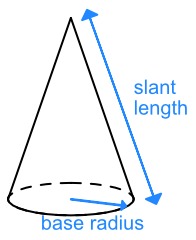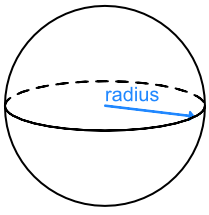Volumes of Circle Shapes

# Volumes of Circle Shapes

GCSE(F), GCSE(H),

The volume of a cone is given by Volume = frac(1)(3) x base area x vertical height, or frac(1)(3)Ah

The base area is that of a circle, πr2The volume of a sphere is given by Volume = frac(4)(3)πr3## Examples

1. A solid wooden cone has a vertical height of 24 cm and a diameter across its base of 6cm. What is the volume of the cone?

The area of the base = πr^2

A = π (frac(3)(2))^2 (divide diameter by 2 for the radius)

A = 7.065

Volume of cone = frac(1)(3)Ah

V = 56.52

2. A globe has a radius of 15cm. What is the volume of the globe?

Answer: 14 130 cm3

Volume of a globe = frac(4)(3)πr^3

V = frac(4)(3) xx π xx 15^3

V = 14130 cm^3^